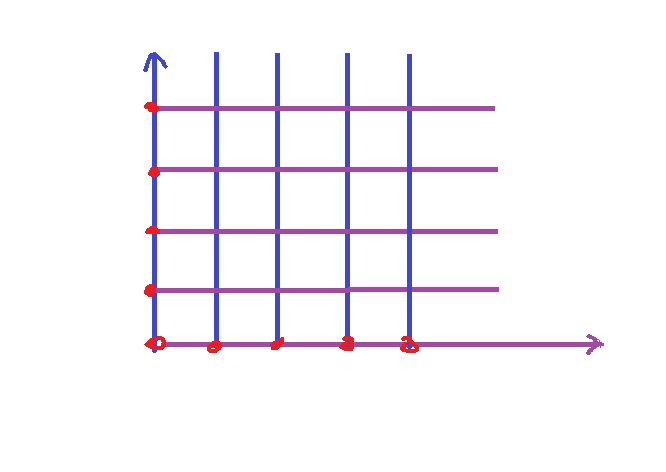3.新的目标：构建更多方块，组成平面

public int xSize =20;
public int zSize =20;vertex count = （xSize+1)*(zSize+1);

vertices = new Vector3[xSize+1)*(zSize+1)];

int i=0;
for (int z=0;z<=zSize;z++)//因为达到最边界zSize也要进行存储计算，所以中间需要计算等于的时候
{
for(int x=0;x<=xSize;x++)
{
vertices[i]=new Vector3(x,0,z);
i++;
//这里需要遍历xSize内元素，引入i这个变量，从而我们可以在开头加入int i=0；由于i在咱们这个z，x循环里使用，我们可以将int i=0；条件放入for(int z=0;这里使得代码更加简练理解，最后代码是
for (int i =0 ,z=0;z<=zSize;z++)
}
}

private void OnDrawGizmos(){
if (vertices==null)
return;
for (int i=0 ;i<vertices.Length;i++)
{
Gizmos.DrawSphere(vertices[i],.1f);
}

triangles =new int;
triangles=0;
triangles=xSize+1;
triangles=1;

triangles=1;
triangles=xSize+1;
triangles=xSize+2;

for (int x=0;x<xSize;x++)
{//将之前6个顶点信息全部剪切进来
triangles =new int;
triangles=0;
triangles=xSize+1;
triangles=1;
triangles=1;
triangles=xSize+1;
triangles=xSize+2;
}

int vert =0;
int tris =0;

    for (int x=0;x<xSize;x++)
{
triangles =new int;
triangles[tris +0]=vert+0;
triangles[tris +1]=vert+xSize+1;
triangles[tris +2]=vert+1;
triangles[tris +3]=vert+1;
triangles[tris +4]=vert+xSize+1;
triangles[tris +5]=vert+xSize+2;

vert++;
tris +=6;
}//第一遍，第一个方块，,tris=0,vert=0,构建完毕后vert+1,第二次循环开始，因为vert=1，此刻进行的是第二个方块顶点存储了。
//同时咱们三角形存储数组大小扩大6格，方便进行第二个方块六个顶点信息存储，
//我们不需要每次循环重复构建存储三角形顶点信息的数组，所以还需要将
triangles =new int;放到for循环外面，在开始并修改元素总数大小为：
triangles =new int[xSize*zSize*6];

Coroutine：协程是让程序员自己作为调度员，告知系统等待某个操作指令完成后再执行后面的代码

void start(){
Debug.Log("start1");
StartCoroutine
(Test());
Debug.Log("start2");
}

IEnumerator Test(){
Debug.Log("test1");
yield return null;
Debug.Log("test2");
}

yield return StartCoroutine(AnotherTest());

IEnumerator AnotherTest(){
Debug.Log("AnotherTest1");
yield return null;
Debug.Log("AnotherTest2");
}

yield return new WaitForSeconds (.1f);

StartCoroutine(CreateShape());

private void Update(){
UpdateMesh();
}

for (int z=0;z<zSize;z++){
将刚才的x轴for循环体全部剪贴进来
}

vert++;

createShape方法里

for(int x=0;x<=xSize;x++)
{
vertices[i]=new Vector3(x,0,z);
i++;

vertices[i]=new Vector3(x,0,z);前增加：

float y =Mathf.PerlinNoise(x*.3f,z*.3f)*2f;//2f使得柏林噪音起伏高低效果更加显著，.3f是形成更广阔的柏林噪音分布，使得地形变化更加多样。

yield return new WaitForSeconds (0.01f);可以修改更快的时间间隔，使得生成的图形更快。

private void OnDrawGizmos()方法，不再需要测试绘制顶点了，保存测试，一张随机全新平面地图快速生成完毕，我们的基本地形生成演示到此完成。

Last modification：August 6, 2020
If you think my article is useful to you, please feel free to appreciate